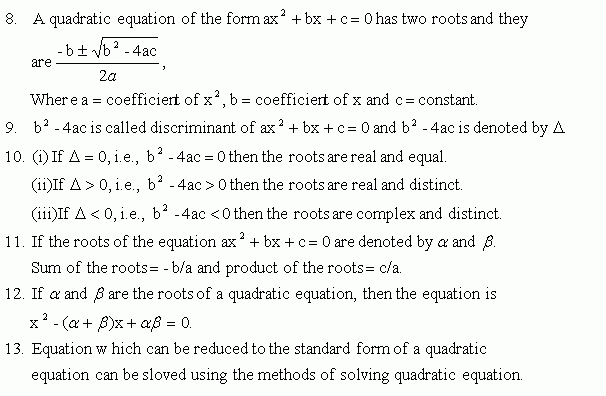Name: ___________________Date:___________________

kwizNET Subscribers, please login to turn off the Ads!
 Email us to get an instant 20% discount on highly effective K-12 Math & English kwizNET Programs!

### High School Mathematics6.8 Square Roots and Algebraic Expressions Review

 1. A square root of x2 is denoted by Öx2 Öx2 = +x or-x. 2. For every x ÎR, x2 ł 0. 3. Ö-5 and Ö -4 do not exist in the set of real number. Square root of a negative number is called an imaginary number. 4. Square roots of algebraic expressions can be found by three methods, they are (i) Inspection Method. (ii) Division Method. (iii) Method of undetermined coefficients. 5. If all the terms of an algebraic expression are of the same degree then such an expression is called a homogeneous expression. 6. An algebraic expression f(x,y) is two variables x and y is said to be a symmetric expression if f(x,y) = f(y,x). 7. Equation of the form ax2 + bx + c = where aą 0, b and cÎ Z is called a Quadratic Equation.Directions: Choose the correct answer.Name: ___________________Date:___________________

### High School Mathematics6.8 Square Roots and Algebraic Expressions Review

 Q 1: Square of the valiable x is _______.Ö x2x2 Q 2: Numbers of the form Ö-6, Ö-5 and Ö-7 are called ________.imaginary numbersReal numbers Q 3: If all the terms of an algebraic expression are of the same degree, then such an expression is called a _______.homogeneous expressionnot a homogeneous expression Q 4: First degree homogeneous expression in x and y is _____.ax + byac * by Q 5: Product of two homogeneous expression is a ________.not a homogeneous expressionhomogeneous expression Q 6: The sum of two symmetric expression is a _______.not a symmetric expressionsymmetric expression Question 7: This question is available to subscribers only! Question 8: This question is available to subscribers only!

#### Subscription to kwizNET Learning System costs less than \$1 per month & offers the following benefits:

• Unrestricted access to grade appropriate lessons, quizzes, & printable worksheets
• Instant scoring of online quizzes
• Progress tracking and award certificates to keep your student motivated
• Unlimited practice with auto-generated 'WIZ MATH' quizzes
• Child-friendly website with no advertisements

© 2003-2007 kwizNET Learning System LLC. All rights reserved. This material may not be reproduced, displayed, modified or distributed without the express prior written permission of the copyright holder. For permission, contact info@kwizNET.com
For unlimited printable worksheets & more, go to http://www.kwizNET.com.